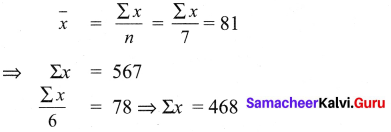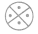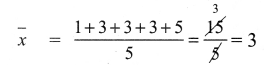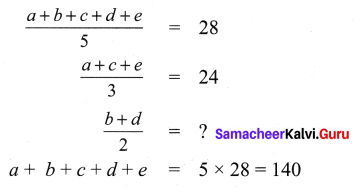# Samacheer Kalvi 9th Maths Solutions Chapter 8 Statistics Ex 8.4

## Tamilnadu Samacheer Kalvi 9th Maths Solutions Chapter 8 Statistics Ex 8.4

Multiple Choice Questions

Question 1.
Let m be the mid point and b be the upper limit of a class in a continuous frequency distribution. The lower limit of the class is
(1) 2 m – b
(2) 2m + b
(3) m – b
(4) m – 2b
Solution:
(1) 2m – bQuestion 2.
The mean of a set of seven numbers is 81. If one of the numbers is discarded, the mean of the remaining numbers is 78 . The value of discarded number is
(1) 101
(2) 100
(3) 99
(4) 98
Solution:
Hint:∴ The discarded number = 567 – 41 = 99
Solution:
(3) 99

Question 3.
A particular observation which occurs maximum number of times in a given data is called its(1) Frequency
(2) range
(3) mode
(4) Median
Solution:
(3) mode

Question 4.
For which set of numbers do the mean, median and mode all have the same values?
(1) 2, 2, 2, 4
(2) 1, 3, 3, 3, 5
(3) 1, 1, 2, 5, 6
(4) 1, 1, 2, 1, 5
Hint:Median = 3
Mode = 3
Solution:
(2) 1, 3, 3, 3, 5Question 5.
The algebraic sum of the deviations of a set of n values from their mean is
(1) 0
(2) n – 1
(3) n
(4) n + 1
Solution:
(1) 0

Question 6.
The mean of a, b, c, d and e is 28 . If the mean of a, c and e is 24, then mean of b and d is
(1) 24
(2) 36
(3) 26
(4) 34
Hint: